Skip to main content

# Quantum Dots

Quantum dots (QDs) are a unique type of nanocrystalline semiconductor whose electronic and optical properties are dependent on the size and shape of the dots. Diameters of these particles can range from about 2-10 nm, on the order of 10-50 atomic lengths6. The small size of the particle gives a high ratio of surface-to-volume, so their properties fall somewhere between that of individual molecules and bulk semiconductors Quantum dots can be single elements (such as silicon, germanium, etc.) or compounds (CdSe, CdS, etc.)6.Figure $$\PageIndex{1}$$: The electronic and optical properties of quantum dots can be fine tuned due to their dependent relationship on size. CdSeqdots" by NASA - mix.msfc.nasa.gov/abstracts.php?p=3906. Licensed under Public Domain via Wikimedia Commons -https://commons.wikimedia.org/wiki/F...:CdSeqdots.jpg

## Theory

Semiconductor crystals of size less than double the Bohr radius of the excitons experience quantum confinement. The particle in a box model can be used to model the energy levels, giving energy states dependent on the size of the potential well2. Three separate scenarios occur7:

• Strong Confinement: The radius of the quantum dot is less than the Bohr radius for both the electron and hole.
• Intermediate Confinement: The radius of the quantum dot is less than the Bohr radius of one of the electron or hole, but not the other.
• Weak Confinement: The radius of the quantum dot is greater than the Bohr radius of both the electron and hole.

The sum of all energies involved with the quantum dots, is expressed by7: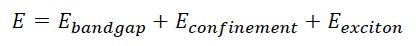This energy is comprised of three terms: band gap energy, confinement energy, and bound exciton energy.

## Band Gap Energy

Band theory in solids refers to the phenomenon in which electrons are known to have ranges of allowed energies (energy bands) and ranges of disallowed energies (forbidden bands or band gaps). Several models such as the Kronig-Penney Model2 and Density Functional Theory2 are used to model band structures in solid state physics.

In the strong confinement scenario, the band gap can increase when the quantum dot is smaller than the exciton bohr radius as energy levels become split4. Total emission energy is increased, as well as emission at varied wavelengths, creating white light.

The exciton Bohr radius is given by the formula7:• εr = dielectric constant (relative permittivity)
• m = mass
• μ = reduced mass
• ab = Bohr radius (0.053 nm)

## Confinement Energy

The particle in a box model is also used in modeling the exciton. Variance of particle size allows for control of the confinement energy. The solution to the particle in a box model is used to represent the energy of the exciton as follows7: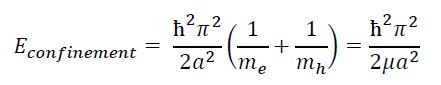## Bound Exciton Energy

Coulombic attractions persist between the electron of negative charge and hole of positive charge that have an energy proportional to Rydberg's energy, and inversely proportional to the dielectric constant squared. This term becomes important when the semiconductor crystal is smaller than the exciton Bohr radius7:A quantum dot is confined in all three spatial dimensions, but semiconductors with other modes of confinement include quantum wires (holes or electrons confined in two spatial dimensions with one degree of freedom), and quantum wells (confined in one spatial dimension with two degrees of freedom).

Taking into account each of these three terms then, the total energy can be simplified further7:## Optical Properties

Wavelengths of light emitted by a quantum dot depends on its size. As the size decreases, the wavelength emitted also shortens, and moves toward the blue end of the visual electromagnetic spectrum2. Conversely, increasing the dot size lengthens the wavelengths emitted, moving toward the red end.

By utilizing the calculated energy along with the deBroglie relationship2: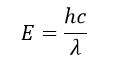A specific wavelength of emitted light can be measured. This implies that quantum dots are highly useful for their allowance to finely tune specific properties such as emitted wavelength.

Fluorescence is generated in quantum dots with an electron is excited from the conduction band to the valence band, and a photon is emitted with a wavelength longer than that absorbed from the recombination of an electron with a hole. The energy difference involved in this process is referred to as the Stokes shift. The emission wavelength and color depend on the size of the quantum dot. Emitted wavelengths range from infrared (IR) to ultraviolet (UV). Since quantum dots of different sizes are able to emit varied wavelengths when excited by a single light source, multiplexing can occur.

## Electronic Properties

Smaller crystals typically have larger electronic bandgaps, meaning that the energetic difference between energy states is greater. This results in more energy being necessary in order to move into an excited state, and more energy is increased upon returning to a rest energy. A semiconductor struck by a photon of light at higher energy that its bandgap will have an electron excited from the valence band to the conduction band (see Figure 2). This process leaves behind a hole, oppositely charged from the electron in the valence band. The hole and electron are attracted by Coulombic forces to create an exciton.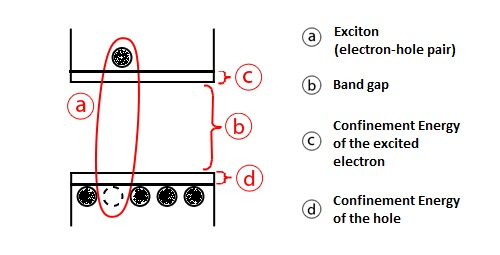Figure 2: Creation of the exciton from the electron hole pair. Exicton energy levels" by Jpailee - Own work. Licensed under CC BY-SA 3.0 via Wikimedia Commons -https://commons.wikimedia.org/wiki/File:Exicton_energy_levels.jpg#/media/File:Exicton_energy_levels.jpg

An exciton is an electrically neutral quasiparticle found in semiconductors, insulators, as well as some liquids. The concept of the exciton was first proposed in 1931 by Yakov Frenkel in a discussion on excitation of atoms within the lattice of insulators.

Brus Equation

The Brus equation is a commonly used method of describing emission energies of quantum dot nanocrystals in terms of the band gap energy, Planck's constant h, the radius of the quantum dot r, and the effective masses of the electron and hole7: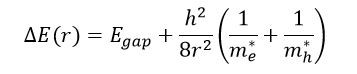## Production

Quantum dots linked together can form the basic building blocks of a variety of crystalline and non-crystalline nanostructures. The quantum dots can be linked together as molecules, more complex structures such as lattices, they can attach to polymeric backbones, and can also be incorporated into thin polymer films5.Figure 3: Quantum dots utilized in a variety of structures, including (a) molecular, (b) crystalline, (c) polymeric, and in (d) thin films.

Techniques for production of quantum dots includes5:

• Colloidal Synthesis
• Electrochemical Assembly
• Fabrication
• Viral Assembly

## Applications

### Quantum Computing

Quantum dots represent one of the more promising candidates for making solid state quantum computing a reality. By utilizing a small voltage applied to the leads, electron flow through the dot can be controlled5. This may allow for precise measurements to be made of properties such as spin. It has been suggested that qubits, or entangled quantum dots, may offer a solution to the quantum computing problem5.

### Medical Imaging

Researchers have found that using latex beads filled with QD nanocrystals can be used to bind to specific DNA sequences5. Probes can be created that combine different sized dots within these beads that emit specific wavelengths and intensities of light. Under UV simulation, these beads serve as markers to identify specific regions within DNA. This process has applications specifically in areas of cancer research.

### Batteries and chargers

Researchers at Vanderbilt University have found that by using quantum dots made of iron pyrite, a modern smartphone can be fully charged within 30 seconds, for dozens of charging cycles4. While the technology is still relatively new, it shows great promise for another highly accessible technological use.

### Solar Energy

Incoming sunlight is absorbed by quantum dots, creating excitons which must be quickly separated5. Electrons travel to the photoanode by metal oxide nanorods, holes to the photocathode by a conducting polymer5. This separation produces a current in the semiconductor. Quantum dots show promising use in producing transparent and semi-transparent windows that are able to act as solar panels.

## Questions

(1) Considering the particle in a box model, the strong confinement scenario occurs when the radius of the quantum dot is:

A) More than the Bohr radius of both the electron and hole.

B) More than the Bohr radius of only one of the electron or hole.

C) Less than the Bohr radius of both the electron and hole.

(2) Using the below information, please determine the emission energy of the photon of light emitted from the CdSe nanocrystals:

Egap = 1.74eV

me* = 0.13me

mh* = 0.45me

r = 5.6nm

(3) Briefly describe an exciton.

### Answers

(1) C

(2) 2.185 (10-18) J or 13.63 eV

(3) An exciton is a bound state of an electron and hole due to Coulombic forces. They can form when a semiconductor absorbs a photon.

## References

1. Brus, L. Chemistry and Physics of Semiconductor Nanocrystals. Web. 8 Dec 2015.
2. Hummel, R. Electronic Properties of Materials, 2nd Edition, New York: Springer-Verlag 1992.
3. Kasap, S.O. Principles of Electronic Materials and Devices, 2nd Edition. New York, NY: McGraw-Hill, 2002.
4. Salisbury, David. Quantum Dots Made From Fool's Gold Boost Battery Performance. Vanderbilt University. Web. 8 Dec 2015.
5. Smith, Yolanda. Quantum Dot Optical Properties. NewsMedical: Life Sciences and Medicine. Web. 8 Dec 2015.
6. Various Authors. Quantum Dots. SigmaAldrich.Web. 8 Dec 2015
7. Various Authors. Quantum Physics. Rutgers University. Web. 8 Dec 2015.
• Was this article helpful?